Create a ggplot with axes set to pixel coordinates and plot the raster image on it using ggplot2::annotation_raster. See examples for how to plot an image onto an existing ggplot.

## Usage

``image_ggplot(image, interpolate = FALSE)``

## Arguments

image

magick image object returned by `image_read()` or `image_graph()`

interpolate

passed to ggplot2::annotation_raster

## Examples

``````# Plot with base R
plot(logo)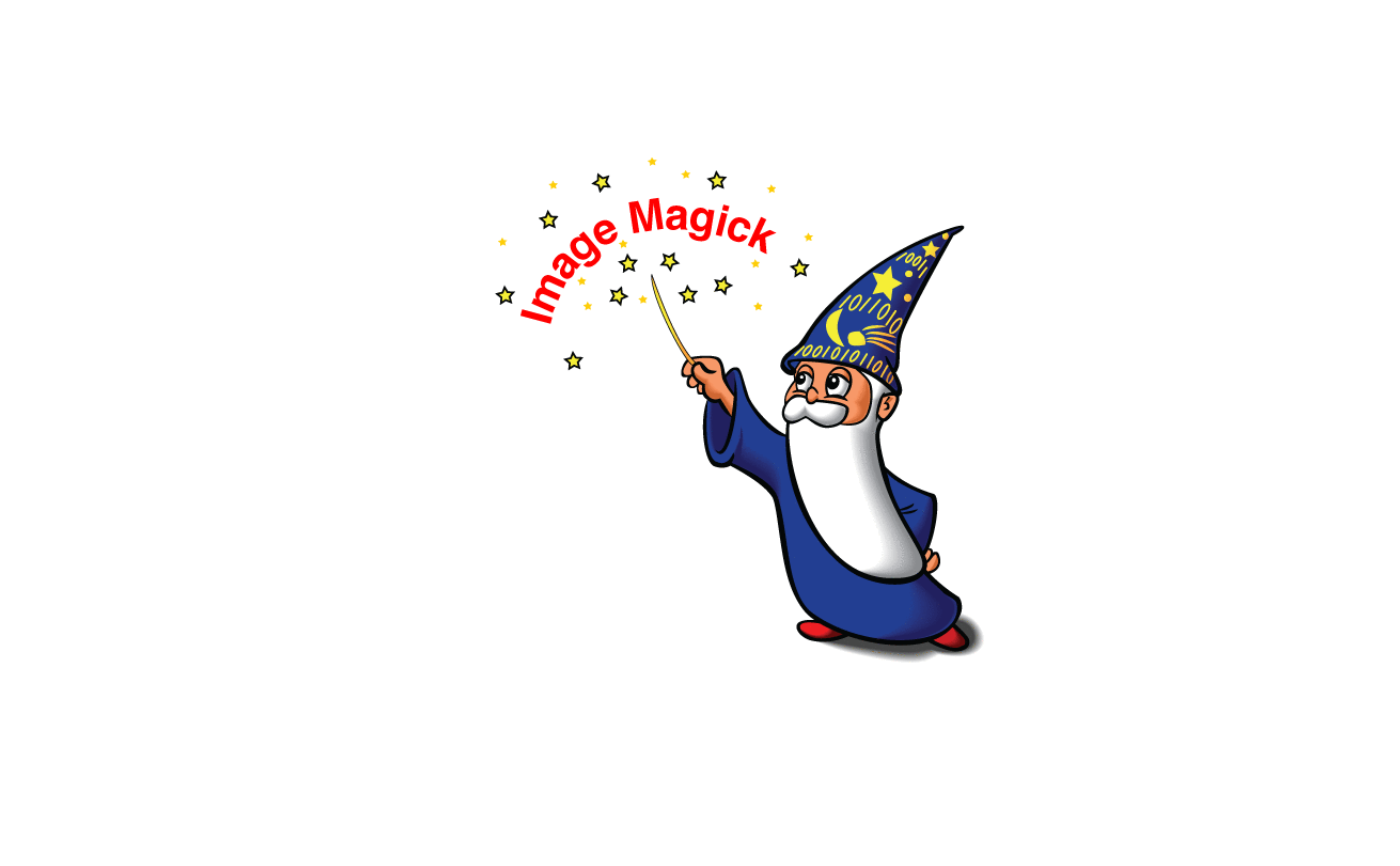# Plot image with ggplot2
library(ggplot2)
myplot <- image_ggplot(logo)
myplot + ggtitle("Test plot")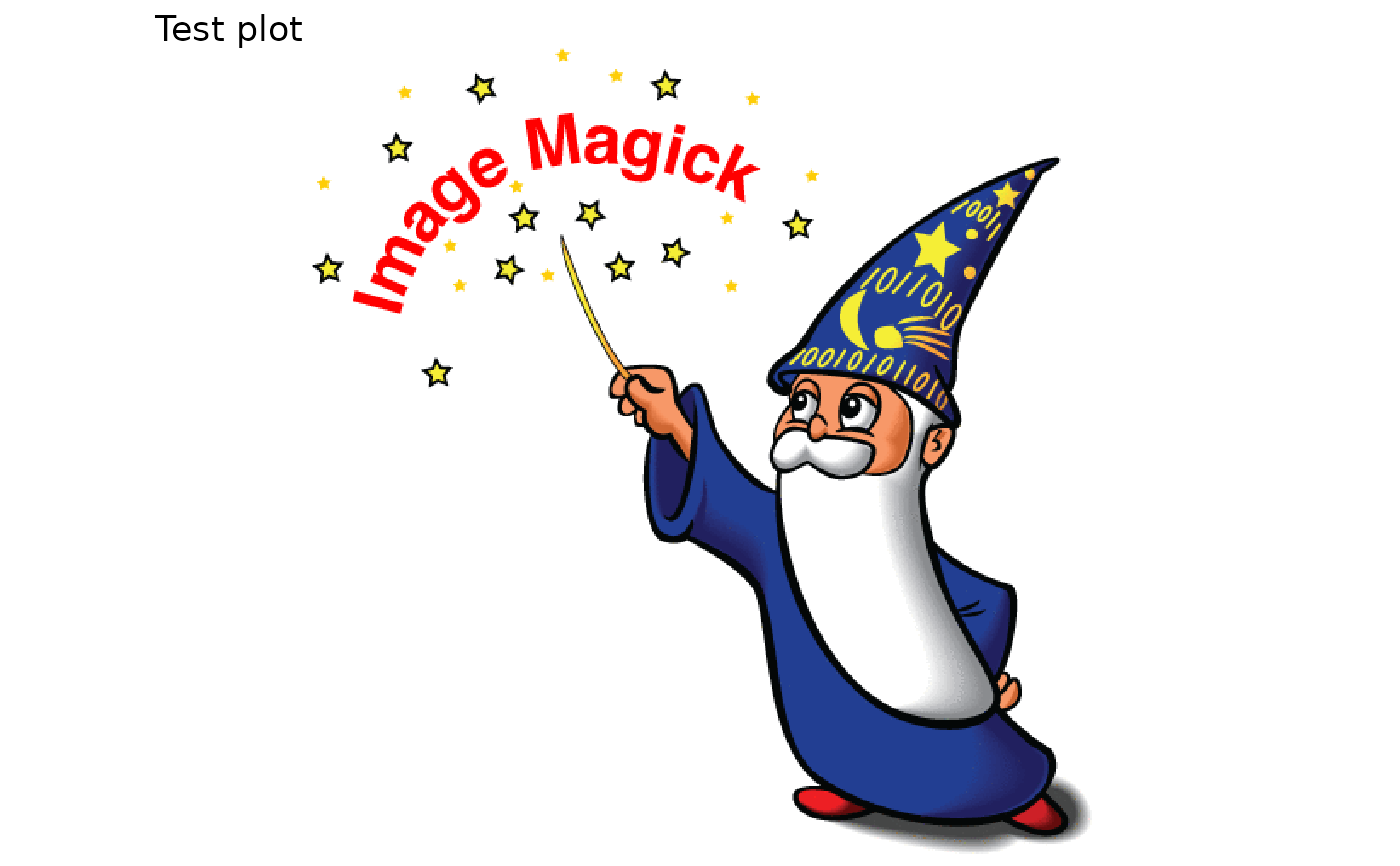# Show that coordinates are reversed:
myplot + theme_classic()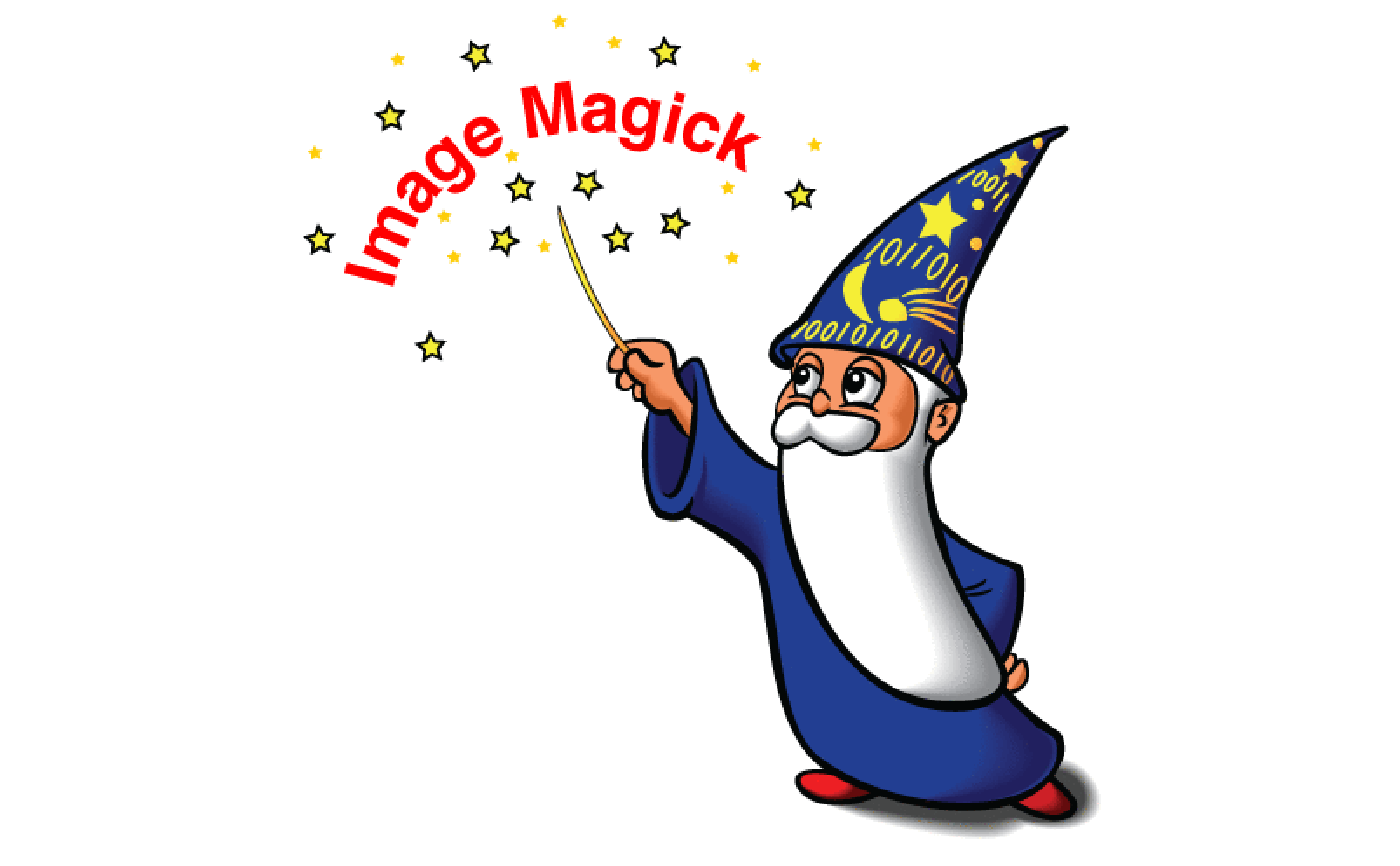# Or add to plot as annotation
image <- image_fill(logo, 'none')
raster <- as.raster(image)
myplot <- qplot(mpg, wt, data = mtcars)
myplot + annotation_raster(raster, 25, 35, 3, 5)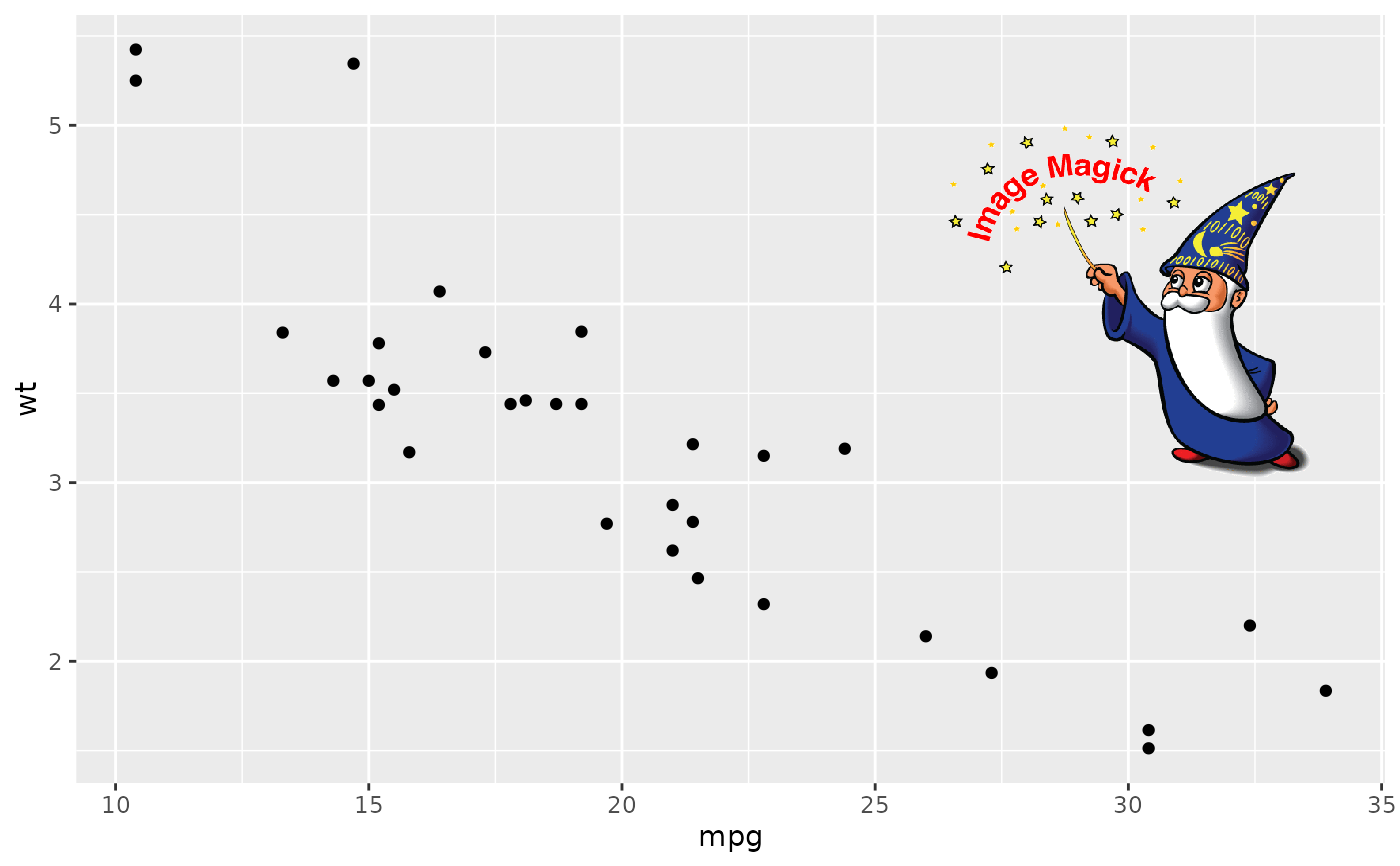# Or overplot image using grid
library(grid)
qplot(speed, dist, data = cars, geom = c("point", "smooth"))
#> `geom_smooth()` using method = 'loess' and formula = 'y ~ x'
grid.raster(image)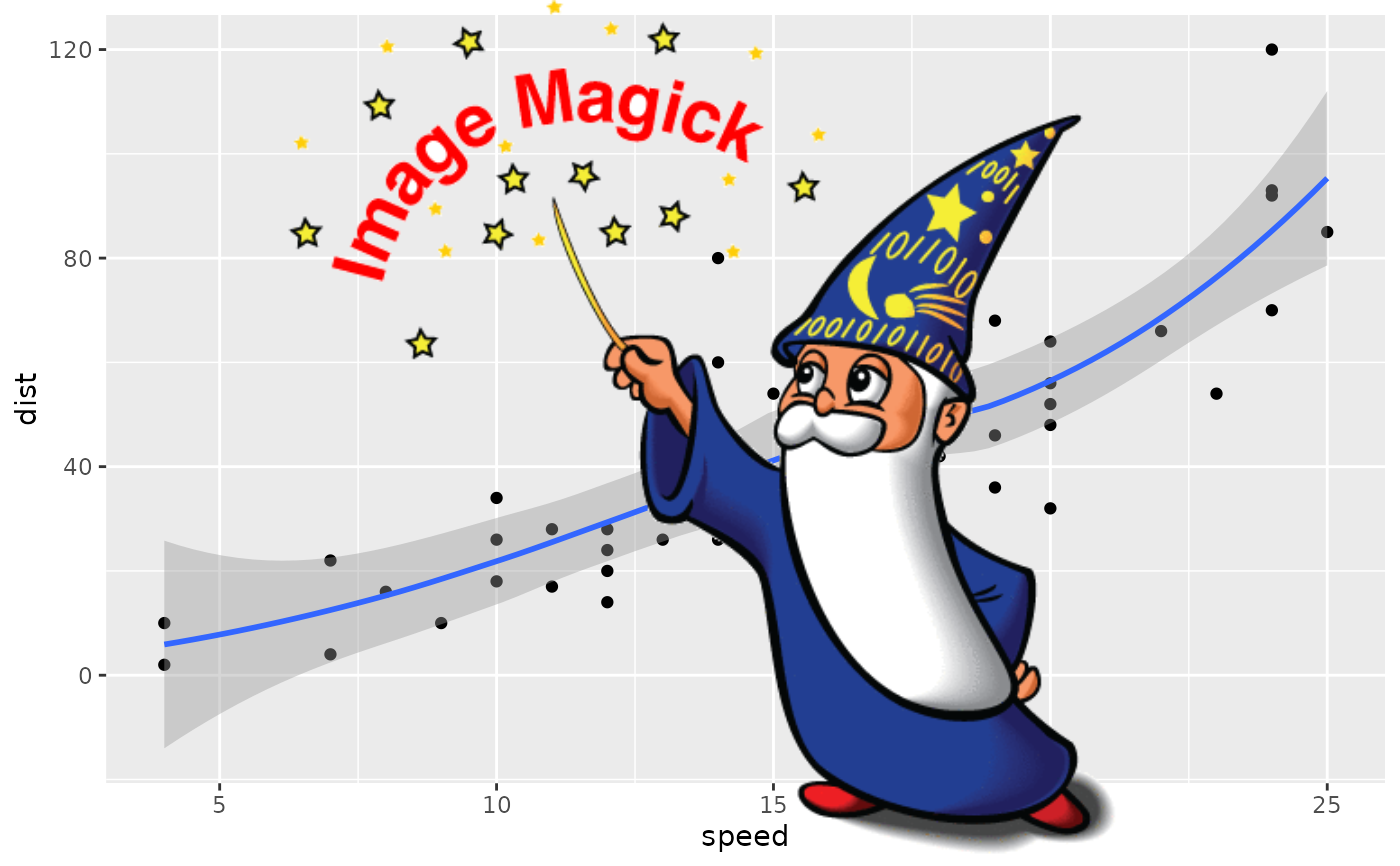``````